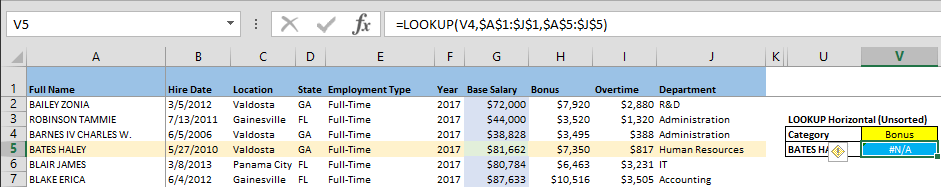There's various functions and combinations of functions that can be used to lookup data in Excel. In this article, we compare the three common formulas used for this job, LOOKUP, VLOOKUP, and HLOOKUP.

# VLOOKUP & HLOOKUP

The famous VLOOKUP is widely used by many professionals building data models in Excel. Its purpose is to search for specific data, and return the corresponding result. This feature comes in very useful when trying to match names, prices, or other features with data from a table. Although not as widely known, the HLOOKUP function does basically the same thing, but in a transposed search pattern.

Both functions look up and find a value within a table selection. While VLOOKUP uses the first column to search for the lookup value (first argument of both functions), HLOOKUP uses the first row. After finding the lookup value in a row or column, both functions return the value specified in the column or row number in table array selection. The very last parameter determines whether the formula should look for an exact or approximate string (range lookup).

Let’s see both formulas in an example. You can download our workbook template pressing the button below.

Searching for the Base Salary of an employee whose name was entered into the corresponding search box, the syntax for these two formulas would be,

=VLOOKUP(Lookup_value, table_array, col_index_num, [range_lookup])

=VLOOKUP(M4,\$A\$1:\$J\$318,7,FALSE)=HLOOKUP(Lookup_value, table_array, row_index_num, [range_lookup])

=HLOOKUP(P4,\$A\$1:\$J\$318,5,FALSE)# LOOKUP

Before its ‘V’ and ‘H’ variants, the plain LOOKUP function was used for looking up calculations and some complex formulas to find unique values in a list. Now, it’s mainly kept in Excel mainly for backward compatibility.

The LOOKUP function searches a value in a single row or column, and returns the value from the same position of another row or column. This behavior is different than how VLOOKUP and HLOOKUP work, and can pose an advantage in some scenarios. Since you don’t need to select the entire table, you don’t need a column or row index to indicate their positions. This property makes the bare form of the LOOKUP formula more flexible than VLOOKUP and HLOOKUP.

A shortcoming of the LOOKUP function is that the lookup array must be sorted in a certain way, otherwise the function won’t be able to find the lookup value and will give “#N/A!” error. VLOOKUP and HLOOKUP on the other hand, have a [range_lookup] parameter that allows you to search in unsorted arrays.

LOOKUP function has two forms: Vector form and Array form. What we described until here was the vector form where you look up a value in a vector (row or column), and return a value from another. Array form is the out-of-date version of VLOOKUP and HLOOKUP functions, and even Microsoft suggests using VLOOKUP and HLOOKUP instead.

The syntax for the vector form of LOOKUP,

=LOOKUP(lookup_value, lookup_vector, [result_vector])

=LOOKUP(S4,\$A\$2:\$A\$318,\$G\$2:\$G\$318)Here is example of how LOOKUP behaves with not sorted data:The formula in cell V5 returns #N/A!, because Category names here are not sorted in alphabetical order.

# Pros and Cons

 VLOOKUP & HLOOKUP LOOKUP Pros Pros Option to search in sorted and unsorted arrays Not affected by inserting, moving and deleting columns or rows Single formula for both vertical and horizontal search Cons Cons Inserting, moving and deleting columns or rows may cause wrong results Returns #N/A with if lookup array is not sorted

Ultimately, all three formulas do the same thing. Their operating logic, however, is something you should keep in mind when choosing which one to use in your data models.